The 14 Different Types of Convex Pentagons that Tile the Plane

All these tilings can be play with online at the Wolfram|Alpha Pentagon Tiling page.

Doris Schattschneider's interactive tiling page is here. Bob Jenkin's half-bath has a beautiful tiling based on the Hirschhorn Medalion. This is one pentagon in the Chaos Tiles. Bob Jenkins has made a page about non-convex pentagons. Marjorie Rice, who discovered many of these, has a page here. A bit more is at MathWorld and Wikipedia. An interactive demonstration of pentagon tilings is available. Some of these might be interesting to study in the context of the Clean Tile Problem, a gambling game with interesting odds and probabilities. This problem is especially interesting If you like to play bingo and other similar games, since it is essentially a betting games based on probable outcomes. Those who play deal or no deal may try to implement something similar but ultimately you can only take your strategy so far.

Most math teachers know that the best way for students to improve at mathematics is for them to regularly practice solving mathematical problems. The challenge that teachers face is how to make practicing the same activity again and again, always seem new, interesting and engaging to students. One technique is to integrate educational games and puzzles into their lessons - provided the games are carefully chosen, students can learn while having fun. Casino games such as blackjack, poker and roulette are a great way to help students calculate simple math; more advance students can discuss the odds and probability of the game.Type 1 : D + E = 180 Type 2 : C + E = 180, a = dType 3 : A = C = D = 120, a = b, d = c + e Type 4 : A = C = 90, a = b, c = d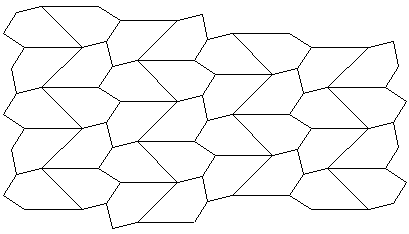Type 5 : C = 2A = 120, a = b, c = d Type 6 : C + E = 180, A = 2C, a = b = e, c = dType 7 : 2B + C = 360, 2D + A = 360, a = b = c = d Type 8 : 2A + B = 360, 2D + C = 360, a = b = c = d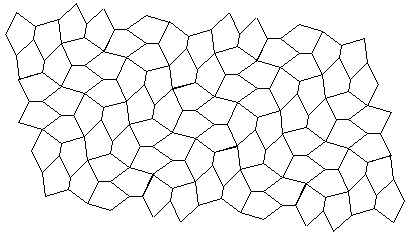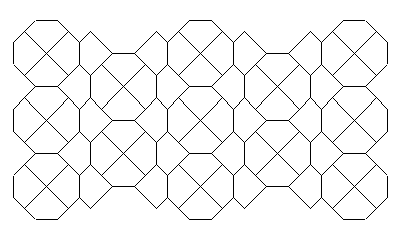Type 9 : 2E + B = 360, 2D + C = 360, a = b = c = d Type 10 : E = 90, A + D = 180, 2B - D = 180, 2C + D = 360, a = e = b + d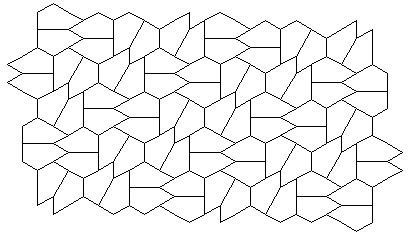Type 11 : A = 90, C + E = 180, 2B + C = 360, d = e = 2a + c Type 12 : A = 90, C + E = 180, 2B + C = 360, 2a = c + e = d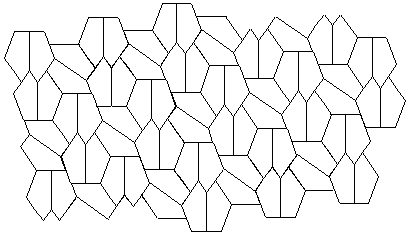Type 13 : A = C = 90, 2B = 2E = 360 - D, c = d, 2c = e Type 14 : D = 90, 2E + A = 360, C + A = 180, B + D + E = 360, 2e = 2c = a

Types 1-5 were found by K. Reinhardt in 1918.
Types 6-8 were found by R. B. Kershner in 1968.
Type 10 was found R. James in 1975.
Types 9, 11-13 were found by M. Rice in 1976-1977.
Type 14 was found by R Stein in 1985.

Sources:
The Penguin Dictionary of Curious and Interesting Geometry, David Wells, 1991.
Mathematical Recreations: A Collection in Honor of Martin Gardner, David Klarner, 1981.
Tilings and Patterns, Branko Grunbaum and G. C. Shephard